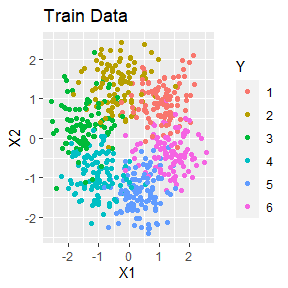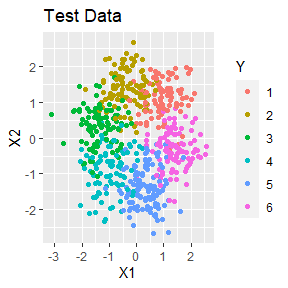# Package Introduction

DANN is a variation of k nearest neighbors where the shape of the neighborhood takes into account training data’s class. The neighborhood is elongated along class boundaries and shrunk in the orthogonal direction. See Discriminate Adaptive Nearest Neighbor Classification by Hastie and Tibshirani. This package implements DANN and sub-DANN in section 4.1 of the publication and is based on Christopher Jenness’s python implementation.

# Arguments

• k - The number of points in the neighborhood. Identical to k in standard k nearest neighbors.
• neighborhood_size - The number of data points used to estimate a good shape for the neighborhood.
• epsilon - Softening parameter. Usually has the least affect on performance.

# Example: Clustered Data

In this example, a simulated data set is made. There is some overlap between classes.

library(dann)
library(dplyr, warn.conflicts = FALSE)
library(ggplot2)
library(mlbench)

set.seed(1)
train <- mlbench.2dnormals(600, cl = 6, r = sqrt(2), sd = .5) %>%
tibble::as_tibble()
colnames(train) <- c("X1", "X2", "Y")
train <- train %>%
mutate(Y = as.numeric(Y))

ggplot(train, aes(x = X1, y = X2, colour = as.factor(Y))) +
geom_point() +
labs(title = "Train Data", colour = "Y")test <- mlbench.2dnormals(600, cl = 6, r = sqrt(2), sd = .5) %>%
tibble::as_tibble()
colnames(test) <- c("X1", "X2", "Y")
test <- test %>%
mutate(Y = as.numeric(Y))

ggplot(test, aes(x = X1, y = X2, colour = as.factor(Y))) +
geom_point() +
labs(title = "Test Data", colour = "Y")To train a model, the data and a few parameters are passed into dann. Neighborhood_size is the number of data points used to estimate a good shape of the neighborhood. K is the number of data points used in the final classification. Considering there is overlap between all classes and there are only about 100 data points per class, dann performs well for this data set.

dannPreds <- dann_df(formula = Y ~ X1 + X2, train = train, test = test,
k = 7, neighborhood_size = 150, epsilon = 1)
round(mean(dannPreds == test\$Y), 2)
#>  0.8